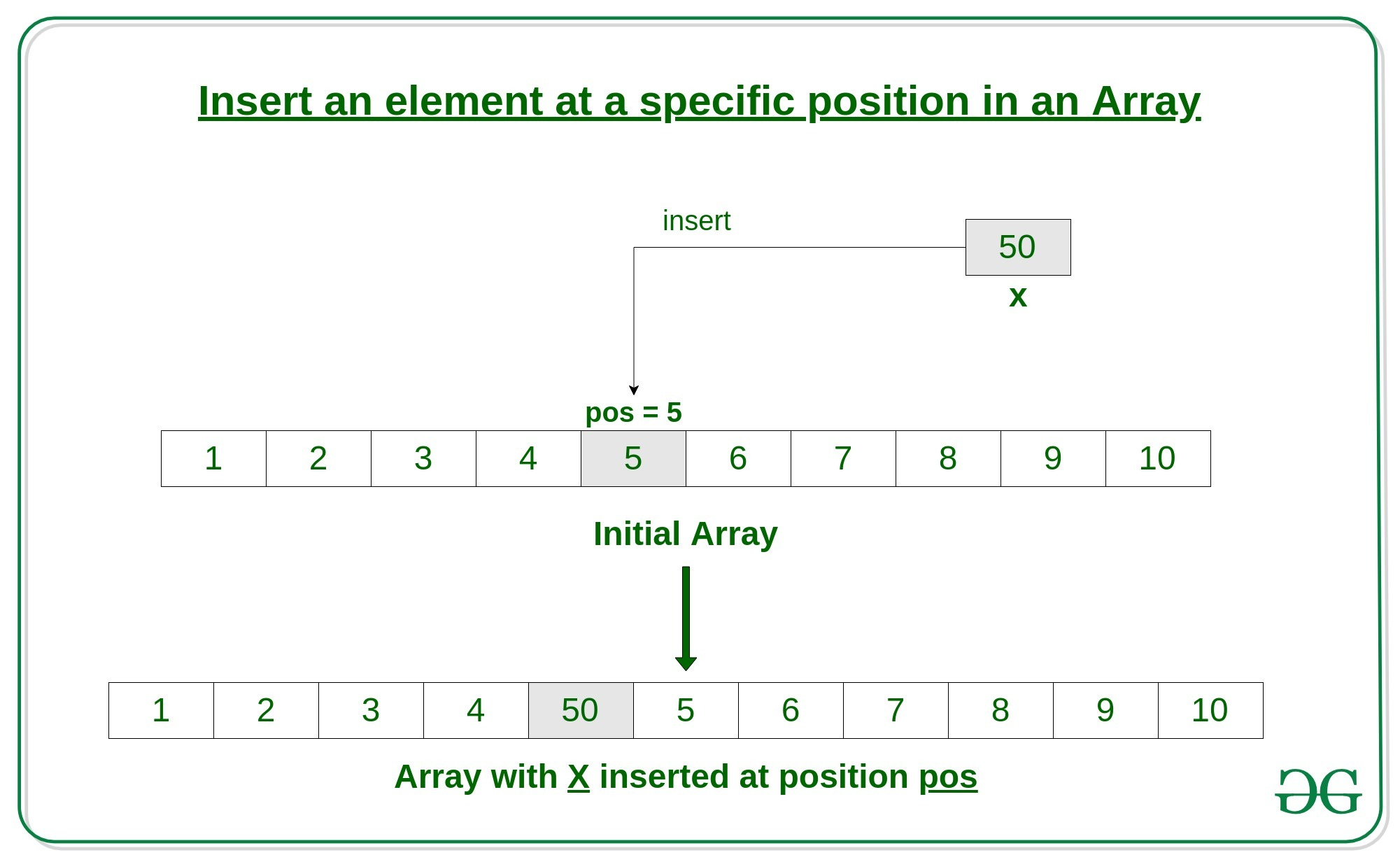# How to Insert an element at a specific position in an Array in Java

An array is a collection of items stored at contiguous memory locations. In this article, we will see how to insert an element in an array in Java.

Given an array arr of size n, this article tells how to insert an element x in this array arr at a specific position pos.Approach 1:
Here’s how to do it.

1. First get the element to be inserted, say x
2. Then get the position at which this element is to be inserted, say pos
3. Create a new array with the size one greater than the previous size
4. Copy all the elements from previous array into the new array till the position pos
5. Insert the element x at position pos
6. Insert the rest of the elements from the previous array into the new array after the pos

Below is the implementation of the above approach:

 `// Java Program to Insert an element ` `// at a specific position in an Array ` ` `  `import` `java.io.*; ` `import` `java.lang.*; ` `import` `java.util.*; ` ` `  `class` `GFG { ` ` `  `    ``// Function to insert x in arr at position pos ` `    ``public` `static` `int``[] insertX(``int` `n, ``int` `arr[], ` `                                ``int` `x, ``int` `pos) ` `    ``{ ` `        ``int` `i; ` ` `  `        ``// create a new array of size n+1 ` `        ``int` `newarr[] = ``new` `int``[n + ``1``]; ` ` `  `        ``// insert the elements from ` `        ``// the old array into the new array ` `        ``// insert all elements till pos ` `        ``// then insert x at pos ` `        ``// then insert rest of the elements ` `        ``for` `(i = ``0``; i < n + ``1``; i++) { ` `            ``if` `(i < pos - ``1``) ` `                ``newarr[i] = arr[i]; ` `            ``else` `if` `(i == pos - ``1``) ` `                ``newarr[i] = x; ` `            ``else` `                ``newarr[i] = arr[i - ``1``]; ` `        ``} ` `        ``return` `newarr; ` `    ``} ` ` `  `    ``// Driver code ` `    ``public` `static` `void` `main(String[] args) ` `    ``{ ` ` `  `        ``int` `n = ``10``; ` `        ``int` `i; ` ` `  `        ``// initial array of size 10 ` `        ``int` `arr[] ` `            ``= { ``1``, ``2``, ``3``, ``4``, ``5``, ``6``, ``7``, ``8``, ``9``, ``10` `}; ` ` `  `        ``// print the original array ` `        ``System.out.println(``"Initial Array:\n"` `                           ``+ Arrays.toString(arr)); ` ` `  `        ``// element to be inserted ` `        ``int` `x = ``50``; ` ` `  `        ``// position at which element ` `        ``// is to be inserted ` `        ``int` `pos = ``5``; ` ` `  `        ``// call the method to insert x ` `        ``// in arr at position pos ` `        ``arr = insertX(n, arr, x, pos); ` ` `  `        ``// print the updated array ` `        ``System.out.println(``"\nArray with "` `+ x ` `                           ``+ ``" inserted at position "` `                           ``+ pos + ``":\n"` `                           ``+ Arrays.toString(arr)); ` `    ``} ` `} `

Output:

```Initial Array:
[1, 2, 3, 4, 5, 6, 7, 8, 9, 10]

Array with 50 inserted at position 5:
[1, 2, 3, 4, 50, 5, 6, 7, 8, 9, 10]
```

Approach 2:
Here’s how to do it.

1. First get the element to be inserted, say element
2. Then get the position at which this element is to be inserted, say position
3. Convert array to ArrayList
5. Convert ArrayList back to array and print

Below is the implementation of the above approach:

 `// Java Program to Insert an element ` `// at a specific position in an Array ` `// using ArrayList ` `import` `java.util.ArrayList; ` `import` `java.util.Arrays; ` `import` `java.util.List; ` ` `  `public` `class` `AddElementAtPositionInArray { ` `     `  `    ``// Mehtod to add element at position ` `    ``private` `static` `void` `addElement( ` `        ``Integer[] arr, ``int` `element, ` `        ``int` `position) ` `    ``{ ` `        ``// Coverting array to ArrayList ` `        ``List list = ``new` `ArrayList<>( ` `            ``Arrays.asList(arr)); ` `         `  `        ``// Adding the element at position ` `        ``list.add(position - ``1``, element); ` `         `  `        ``// Converting the list back to array ` `        ``arr = list.toArray(arr); ` ` `  `        ``// Printing the original array ` `        ``System.out.println(``"Initial Array:\n"` `                        ``+ Arrays.toString(arr)); ` ` `  `        ``// Printing the updated array ` `        ``System.out.println(``"\nArray with "` `+ element ` `                        ``+ ``" inserted at position "` `                        ``+ position + ``":\n"` `                        ``+ Arrays.toString(arr)); ` `    ``} ` `     `  `    ``// Drivers Method ` `    ``public` `static` `void` `main(String[] args) ` `    ``{ ` `        ``// Sample array ` `        ``Integer[] arr = { ``1``, ``2``, ``3``, ``4``, ``5``, ` `                        ``6``, ``7``, ``8``, ``9``, ``10` `}; ` `     `  `        ``// Element to be inserted ` `        ``int` `element = ``50``; ` `     `  `        ``// Position to insert ` `        ``int` `position = ``5``; ` `     `  `        ``// Calling the function to insert ` `        ``addElement(arr, element, position); ` `    ``} ` `} `

Output:

```Initial Array:
[1, 2, 3, 4, 5, 6, 7, 8, 9, 10]

Array with 50 inserted at position 5:
[1, 2, 3, 4, 50, 5, 6, 7, 8, 9, 10]
```

My Personal Notes arrow_drop_upCheck out this Author's contributed articles.

If you like GeeksforGeeks and would like to contribute, you can also write an article using contribute.geeksforgeeks.org or mail your article to contribute@geeksforgeeks.org. See your article appearing on the GeeksforGeeks main page and help other Geeks.

Please Improve this article if you find anything incorrect by clicking on the "Improve Article" button below.

Improved By : GauravWable

Article Tags :

Be the First to upvote.

Please write to us at contribute@geeksforgeeks.org to report any issue with the above content.